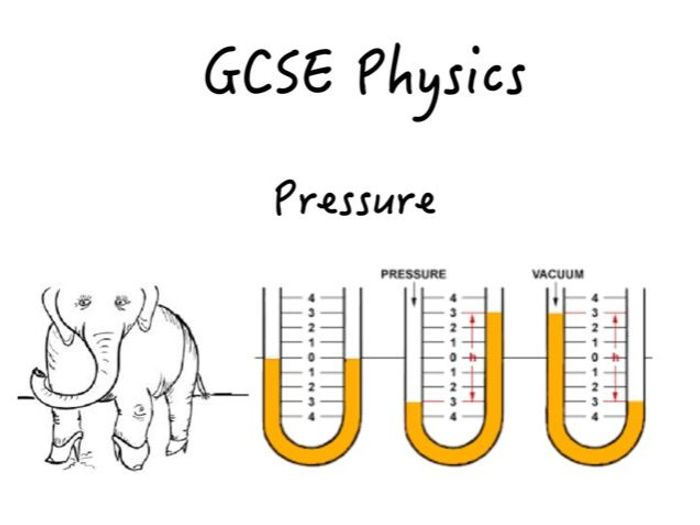A booklet of notes, activities, calculations, exam technique in answering worded questions, practical skills and command word practice on the topic of pressure.

The learning objectives include:

To define the term ‘pressure’
To recall and use the formula for calculating pressure
To describe an experiment to determine the pressure a person exerts on the floor
To understand the pressure at a point in a fluid acts equally in all directions
To recall and use the equation linking pressure difference, density, g and depth
To explain how pressure changes with depth and link this to situations
To explain how a barometer works and use it to calculate pressure
To explain how a manometer works and use it to calculate pressure
To explain why different liquids are used in barometers and manometers

\$5.41
Save for later

### Info

Created: Nov 3, 2019

Updated: Nov 14, 2019

pptx, 752 KB

docx, 5 MB

Pressure-Booklet-TES

pdf, 3 MB

Pressure-Booklet-TES

Report a problem